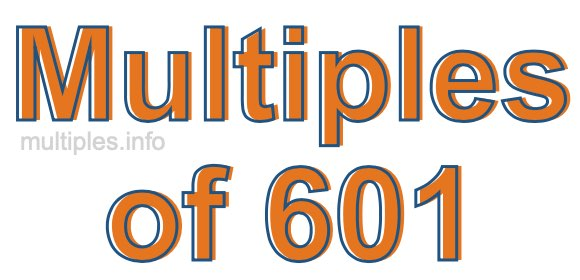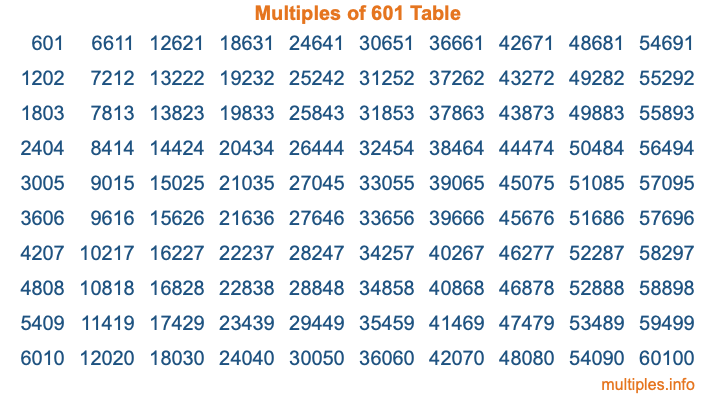Multiples of 601Welcome to the Multiples of 601 page. Here we will first teach you everything you will ever need to know about the multiples of 601, and then give you a study guide summary of everything we taught you to make sure you remember it all. Use this page to look up facts and learn information about the multiples of 601. This page will make you a multiples of six hundred one expert!

Definition of Multiples of 601
Multiples of 601 are all the numbers that when divided by 601 equal an integer. Each of the multiples of 601 are called a multiple. A multiple of 601 is created by multiplying 601 by an integer.

Therefore, to create a list of multiples of 601, you start with 1 multiplied by 601, then 2 multiplied by 601, then 3 multiplied by 601, and so on for as long as you want. Thus, the list of the first five multiples of 601 is 601, 1202, 1803, 2404, and 3005. To see a larger list of multiples of 601, see the printable image of Multiples of 601 further down on this page. We also have a category where you can choose any nth multiple of 601.

Multiples of 601 Checker
The Multiples of 601 Checker below checks to see if any number of your choice is a multiple of 601. In other words, it checks to see if there is any number (integer) that when multiplied by 601 will equal your number. To do that, we divide your number by 601. If the the quotient is an integer, then your number is a multiple of 601.

Is  a multiple of 601?

Least Common Multiple of 601 and ...
A Least Common Multiple (LCM) is the lowest multiple that two or more numbers have in common. This is also called the smallest common multiple or lowest common multiple and is useful to know when you are adding our subtracting fractions. Enter one or more numbers below (601 is already entered) to find the LCM.

Check out our LCM Calculator if you need more details about the Least Common Multiple or if you need the LCM for different numbers for adding and subtraction fractions.

nth Multiple of 601
As we stated above, 601 is the first multiple of 601, 1202 is the second multiple of 601, 1803 is the third multiple of 601, and so on. Enter a number below to find the nth multiple of 601.

th multiple of 601

Multiples of 601 vs Factors of 601
601 is a multiple of 601 and a factor of 601, but that is where the similarities end. All postive multiples of 601 are 601 or greater than 601. All positive factors of 601 are 601 or less than 601.

Below is the beginning list of multiples of 601 and the factors of 601 so you can compare:

Multiples of 601: 601, 1202, 1803, 2404, 3005, etc.

Factors of 601: 1, 601

As you can see, the multiples of 601 are all the numbers that you can divide by 601 to get a whole number. The factors of 601, on the other hand, are all the whole numbers that you can multiply by another whole number to get 601.

It's also interesting to note that if a number (x) is a factor of 601, then 601 will also be a multiple of that number (x).

Multiples of 601 vs Divisors of 601
The divisors of 601 are all the integers that 601 can be divided by evenly. Below is a list of the divisors of 601.

Divisors of 601: 1, 601

The interesting thing to note here is that if you take any multiple of 601 and divide it by a divisor of 601, you will see that the quotient is an integer.

Multiples of 601 Table
Below is an image of the first 100 multiples of 601 in a table. The table is in chronological order, column by column. The first column has the first ten multiples of 601, the second column has the next ten multiples of 601, and so on.The Multiples of 601 Table is also referred to as the 601 Times Table or Times Table of 601. You are welcome to print out our table for your studies.

Negative Multiples of 601
Although not often discussed or needed in math, it is worth mentioning that you can make a list of negative multiples of 601 by multiplying 601 by -1, then by -2, then by -3, and so on, to get the following list of negative multiples of 601:

-601, -1202, -1803, -2404, -3005, etc.

Multiples of 601 Summary
Below is a summary of important Multiples of 601 facts that we have discussed on this page. To retain the knowledge on this page, we recommend that you read through the summary and explain to yourself or a study partner why they hold true.

There are an infinite number of multiples of 601.

A multiple of 601 divided by 601 will equal a whole number.

601 divided by a factor of 601 equals a divisor of 601.

The nth multiple of 601 is n times 601.

The largest factor of 601 is equal to the first positive multiple of 601.

601 is a multiple of every factor of 601.

601 is a multiple of 601.

A multiple of 601 divided by a divisor of 601 equals an integer.

601 divided by a divisor of 601 equals a factor of 601.

Any integer times 601 will equal a multiple of 601.

Multiples of a Number
Here you can get the multiples of another number, all with the same attention to detail as we did for multiples of 601 on this page.

Multiples of
Multiples of 602
Did you find our page about multiples of six hundred one educational? Do you want more knowledge? Check out the multiples of the next number on our list!